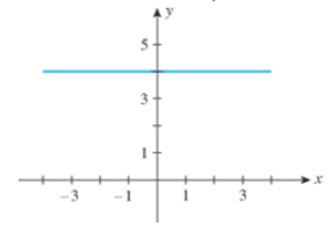Chapter 1.4, Problem 10E

Chapter
Section
Textbook Problem

In Exercises 7-10, find the slope of the line shown in each figure.10.To determine

To find: The slope of the line of the given figure.

Explanation

The graph shows the slope of line,

Figure (1)

The two points on the graph are (0,5) and (3,5) .

The slope of the equation of line is calculated as,

m=y2y1x2x1 (1)

Substit

Still sussing out bartleby?

Check out a sample textbook solution.

See a sample solution

The Solution to Your Study Problems

Bartleby provides explanations to thousands of textbook problems written by our experts, many with advanced degrees!

Get Started

Find more solutions based on key concepts